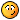# Interior and font color change based on value

S

#### Shu of AZ

I placed a question on General Questions with no resolve and Im hoping to
find it on this board.

Problem: I need to affect a color change in cells that are in a range of 12
cells
based on an auto-populated value.

Criteria:
Cell range o528
(Why the range is larger than 12 is that the cells are merged to equate to
only 12 cells, o5-o6, o7-o8, o9-o10 etc.)

In the workbook there are 12 identically formatted sheets that have this
range on them. Each sheet has a different name. R1, R2, R3, etc.

In the workbook there is also 1 data source sheet that the 12 sheets feed
from to get the cell value from. The value is dumped to the 12 sheets then
using a Vlookup formula in each cell of the range, the cells get their value.

The value that is auto-populated is always 1 thru 12 ( o5 could be 11 and o7
could be 7 and so on. ) Sometimes they are blank.

EXCEPTION: The user cannot manually enter the value or can they press enter
to effect and Event.

EXCEPTION: The user cannot hit enter to cause the change. The event coding
using Case did not work well.

G

#### Gord Dibben

Private Sub Worksheet_Calculate()
End Sub

Gord Dibben MS Excel MVP

R

#### RyanH

You don't have to use VBA for this. If the Range is always O5:O28 then just
highlight the range and select conditional formatting.

Hope this helps!

S

#### Shu of AZ

there are 12 possiblities and only 3 conditionals available

S

#### Shu of AZ

12 different colors too

RyanH said:
You don't have to use VBA for this. If the Range is always O5:O28 then just
highlight the range and select conditional formatting.

Hope this helps!

R

#### RyanH

Ok, just as a word of advise. Merging cells and then coding can kinda be a
pain in the "A". I think this will work for you though. This procedure will
run each time a value is changed in your data source (assumed to be in
Sheet1). It will step thru all worksheets except for Sheet1. In my Select
Case statement I merely made an example to test the code, but you will have
to set your parameters. Paste this code in Worksheet 1 Module using the
Change Event.

Private Sub Worksheet_Change(ByVal Target As Range)

Dim Range1 As Range
Dim Range2 As Range
Dim Range3 As Range
Dim Range4 As Range
Dim Range5 As Range
Dim Range6 As Range
Dim Range7 As Range
Dim Range8 As Range
Dim Range9 As Range
Dim Range10 As Range
Dim Range11 As Range
Dim Range12 As Range
Dim i As Byte
Dim myRanges As Collection
Dim rng As Range

Set Range1 = Range("O5")
Set Range2 = Range("O7")
Set Range3 = Range("O9")
Set Range4 = Range("O11")
Set Range5 = Range("O13")
Set Range6 = Range("O15")
Set Range7 = Range("O17")
Set Range8 = Range("O19")
Set Range9 = Range("O21")
Set Range10 = Range("O23")
Set Range11 = Range("O25")
Set Range12 = Range("O27")

Set myRanges = New Collection
With myRanges
End With

For i = 2 To Worksheets.Count
MsgBox i
For Each rng In myRanges
Select Case rng.Value
Case Is < 3
rng.Interior.Color = vbRed
Case Is = 3
rng.Interior.Color = vbYellow
Case Is > 4
rng.Interior.Color = vbCyan
End Select
Next rng
Next i
End Sub

Hope this helps! If so, please click "yes" below.